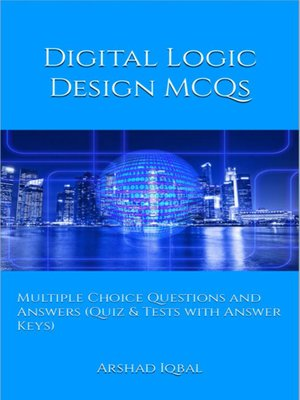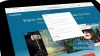# Digital Logic Design MCQs

### Multiple Choice Questions and Answers (Quiz & Tests with Answer Keys)

ebook

With an OverDrive account, you can save your favorite libraries for at-a-glance information about availability. Find out more about OverDrive accounts.

### Subjects

"Digital Logic Design MCQs: Multiple Choice Questions and Answers (Quiz & Tests with Answer Keys)" provides practice tests for competitive exams preparation. "Digital Logic Design MCQ" helps with theoretical, conceptual, and analytical study for self-assessment, career tests. This book can help to learn and practice "Digital Logic Design" quizzes as a quick study guide for placement test preparation.
Digital Logic Design Multiple Choice Questions and Answers pdf is a revision guide with a collection of trivia questions to fun quiz questions and answers pdf on topics: Algorithmic state machine, asynchronous sequential logic, binary systems, Boolean algebra and logic gates, combinational logics, digital integrated circuits, DLD experiments, MSI and PLD components, registers counters and memory units, simplification of Boolean functions, standard graphic symbols, synchronous sequential logics to enhance teaching and learning.
Digital Logic Design Quiz Questions and Answers pdf also covers the syllabus of many competitive papers for admission exams of different universities from computer science textbooks on chapters:

Algorithmic State Machine Multiple Choice Questions: 50 MCQs. Asynchronous Sequential Logic Multiple Choice Questions: 50 MCQs. Binary Systems Multiple Choice Questions: 50 MCQs. Boolean Algebra and Logic Gates Multiple Choice Questions: 50 MCQs. Combinational Logics Multiple Choice Questions: 50 MCQs. Digital Integrated Circuits Multiple Choice Questions: 50 MCQs. DLD Experiments Multiple Choice Questions: 150 MCQs. MSI and PLD Components Multiple Choice Questions: 50 MCQs. Registers Counters and Memory Units Multiple Choice Questions: 50 MCQs. Simplification of Boolean Functions Multiple Choice Questions: 50 MCQs. Standard Graphic Symbols Multiple Choice Questions: 50 MCQs. Synchronous Sequential Logics Multiple Choice Questions: 50 MCQs.

The chapter "Algorithmic State Machine MCQs" covers topics of introduction to algorithmic state machine, algorithmic state machine chart, ASM chart, control implementation in ASM, design with multiplexers, state machine diagrams, and timing in state machines.
The chapter "Asynchronous Sequential Logic MCQs" covers topics of introduction to asynchronous sequential logic, analysis of asynchronous sequential logic, circuits with latches, design procedure of asynchronous sequential logic, and transition table.
The chapter "Binary Systems MCQs" covers topics of binary systems problems, complements in binary systems, character alphanumeric codes, arithmetic addition, binary codes, binary numbers, binary storage and registers, code, decimal codes, definition of binary logic, digital computer and digital system, error detection code, gray code, logic gates, number base conversion, octal and hexadecimal numbers, radix complement, register transfer, signed binary number, subtraction with complement, switching circuits, and binary signals.
The chapter "Boolean Algebra and Logic Gates MCQs" covers topics of basic definition of Boolean algebra, digital logic gates, axiomatic definition of Boolean algebra, basic algebric manipulation, theorems and properties of Boolean algebra, Boolean functions, complement of a function, canonical and standard forms, conversion between canonical forms, standard forms, integrated circuits, logical operations, operator precedence, product of maxterms, sum of minterms, and Venn diagrams.
The chapter "Combinational Logics MCQs" covers topics of introduction to combinational logics, full adders in combinational logics, design procedure in combinational logics, combinational logics analysis procedure, adders, Boolean functions implementations, code conversion,...

### Publication Details

Publisher:
Imprint:
Smashwords Edition
Publication Date:
2016

### Format